### 前言

2023/03/31修改，增加top-p，删掉多余的话，本不打算继续这个系列，但语言生成策略是个通用的东西，还是写完整吧。

### 随机采样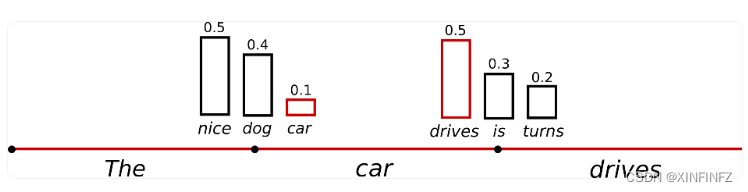``````from transformers import AutoModelForCausalLM, AutoTokenizer, GenerationConfig
import time
a1 = time.time()
checkpoint = "bigscience/bloom-1b1"

tokenizer = AutoTokenizer.from_pretrained(checkpoint)
model = AutoModelForCausalLM.from_pretrained(checkpoint)
inputs = tokenizer.encode("我和我的猫都很想你", return_tensors="pt") #prompt
outputs = model.generate(inputs,min_length=150,
do_sample=True,max_length=200,temperature=1.0)
print(tokenizer.decode(outputs)) #使用tokenizer对生成结果进行解码
a2 = time.time()
print(f'time cost is {a2 - a1} s')
``````

``````我和我的猫都很想你,你却不在,我知道你也在等我,我有些伤感,

time cost is 29.499494075775146 s
``````

### 温度设定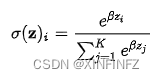``````outputs = model.generate(inputs,min_length=150,do_sample=True,
max_length=200,temperature=0.001)
``````

``````我和我的猫都很想你。 猫咪:你什么时候回来？

time cost is 35.493085861206055 s
``````

``````outputs = model.generate(inputs,min_length=150,do_sample=True,
max_length=200,temperature=100)
``````

T=100，此时β接近于0，概率分布接近于{1/n,1/n,1/n…1/n}，所有词都含有相同被选中的概率，生成的文字结果如下：

``````我和我的猫都很想你:猫小狗喐呔猫 小白兔子,

time cost is 35.58598041534424 s
``````

``````我和我的猫都很想你，因为你是我的偶像。

``````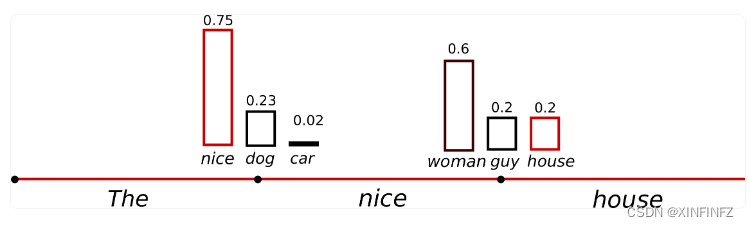### Top-k和Top-p

Top-k就是选取在概率分布里前K个可能性从高到低排序的词，比如top_k=50就是选前五十个词，其他就忽略了。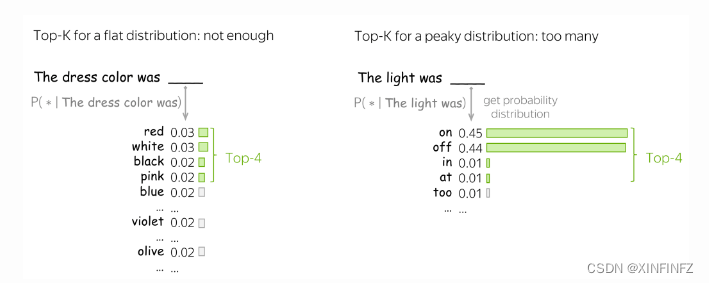Top-p就是选取前N个词的可能性加起来超过设定值，比如top-p=0.95，第一个词概率是0.6，第二个词是0.35，那就只选取前两个词了，后面的会忽略不计。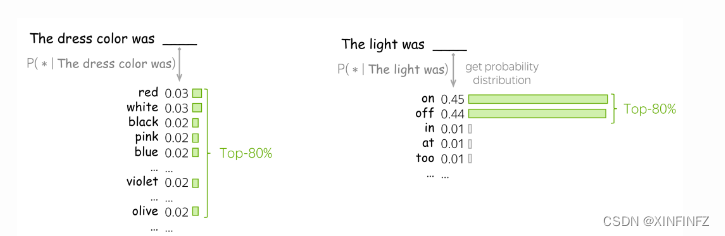``````outputs = model.generate(inputs,min_length=150,do_sample=True,
no_repeat_ngram_size=2,max_length=200,top_p=0.8)
``````

``````我和我的猫都很想你。 在你最需要的时候,你总是会出现在我身边。

``````